Related Articles
Cartesian Coordinate System
• Last Updated : 21 Feb, 2021

A number line can be used to represent a number or solution of an equation that only has one variable. It is sufficient to describe the solution of one-valued equations because they all are single-dimensional. But as the number of variables in an equation increases, it is not enough. For example when the number of variables in an equation becomes two, there will be pair of numbers as a solution. This is why the concept of the number line has to be extended. There should be 2 number lines now, but how will we show our solution on it?

So, instead of a line, let’s define a plane to plot the solutions now.

### Cartesian Plane, Coordinates, and Lines

Cartesian Plane:

A Cartesian plane is defined by two perpendicular number lines, X and Y. It extends to infinity in both directions. It has a center usually denoted by O.

The horizontal line is called X-axis while the vertical line is called Y-axis.

Cartesian Coordinates:

Cartesian Coordinates are used to mark the plane about a point. How far up/down or how far left/right it is.

They are always written in a certain order:

• Horizontal distance
• Vertical Distance

This is called an “ordered pair” (a pair of numbers in a special order) and usually, the numbers are separated by a comma, and parentheses are put around the whole thing like (5,4).

Abscissa and Ordinate:

They are just different names for the x and y values:

• Abscissa: The x-value in a pair of coordinates.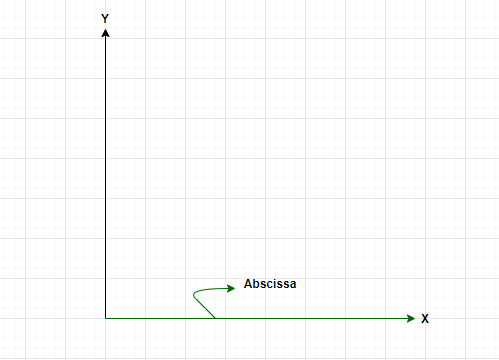• Ordinate: The y-value in a pair of coordinates.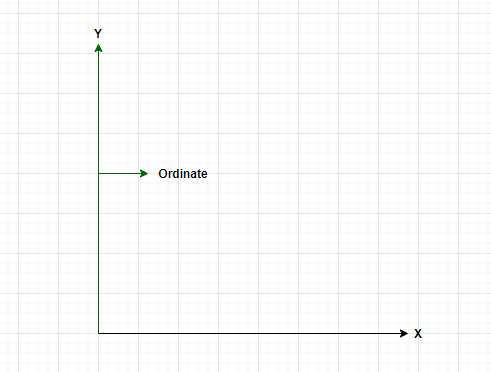Question 1: How much is the distance of the point A (5,4) from the X- Axis?

Point A (5,4) on the XY Plane is lying in such a way that it is 5 Units away from the Y Axis and 4 Units away from the X Axis.

Therefore, Point A (5,4) is 4 Units of distance away from X Axis.

Question 2: How much the distance of the point B (54, 36) from Y-Axis?

Point B (54,36) is lying on the XY Plane. It is clear that point B is 54 units away from the Y Axis and 36 Units away from the X Axis.

Hence, Point B (54, 36) is 54 Units away from Y axis.

### Linear Equation in two Variables

A linear equation in two variables can be expressed to be,

Ax + By = C,

where A, B are not equal to zero.

These equations have more than one solution.

For example: x + 2y = 6

x = 2 and y = 2 satisfy this equation. Similarly, (0,3) is also a solution. There are infinitely many solutions like this. All the points satisfying this equation lie on a straight line. It means that the equations in two variables represent a line on the Cartesian plane.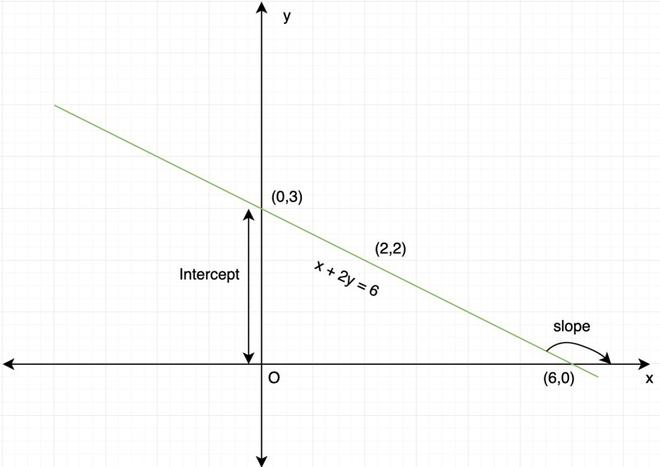All the points satisfying the equation x + 2y = 6 form a line.

A linear equation in two variables can also be written in slope-intercept form to make it easier to plot and interpret on the graph.  The point where a line intersects the y-axis is called intercept. It can be found by putting x = 0 and finding out the “y” through the single variable equation. The angle made by line with the positive x-axis is called the slope.

### Slope Intercept Form

Usually, a linear equation in two variables is written in this form as this is the easiest way to find the slope of the line representing the equation while drawing the graph for it.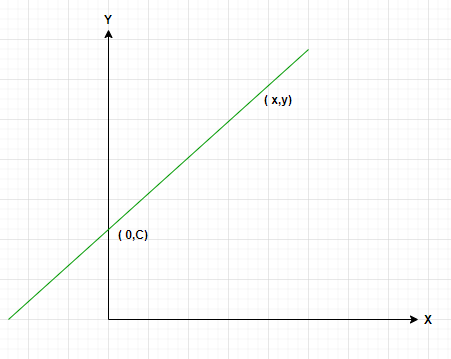Slope Intercept Form

• (0, C) = Intercept of Y- Axis.
• (x, y) = A Point on the line.

The slope-intercept form is:

Y = mX + C

where ‘m’ is the slope of the line and ‘C’ is the intercept(point of intersection of the line with the y-axis).

Note: If the intercept ‘C’ is zero, then the equation of the lines becomes y = mx and it passes through origin.

Question: Plot the line 3x + 2y = 6 on graph.

This equation must be reduced into the slope intercept form so that we can draw this on graph.

3x + 2y = 6

2y = 6 – 3x

⇒ y= 3 – (3/2)x

⇒ y = -(3/2)x + 3

Now this equation can be plotted on graph.

Here, intercept ‘c’ = 3 and slope ‘m’ = -(3/2)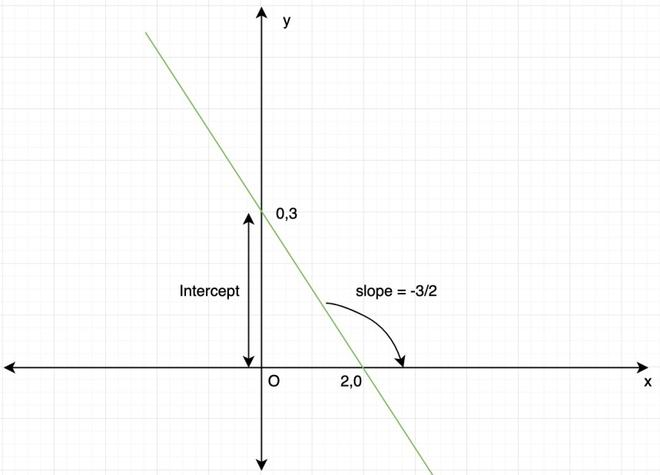Point Slope Form:

It is used to describe the line when slope ‘m’ and one point of the line is available to us.

y – y1 = m(x – x1)

Intercept Form:

Used to describe the line when both x and y-axis intercept are available.Two Point Form:

It is used when two points satisfying the equation of lines are available.### Equations of lines parallel to the x-axis or y-axis

To find the equations of the lines parallel to the x-axis or y-axis.

Let’s say there is a line XY that is parallel to the x-axis and is at a distance of “5” from the x-axis. This means that all the points of the line are 5 units away from the x-axis. Thus, all the points on the line XY satisfy one condition i.e they are all 5 units away from the x-axis.

Let (x, y) be any point on the line XY, then it should satisfy, y = 5.

So, all the lines which are parallel to the x-axis will have a form of y = c where ‘c’ is the distance of the line from the x-axis.

Similarly, all the lines which are parallel to the y-axis will have a form of x = c, where ‘c’ is the distance of the line from the y-axis.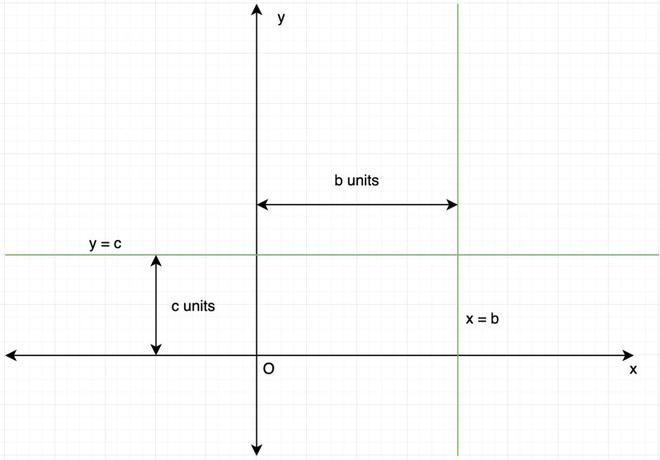### System of Linear Equations

A system of linear equations is formed when two or more linear equations work together. Since each equation represents a line on the Cartesian plane. Geometrically, finding out the solution to the system means finding out a point that satisfies both the lines i.e finding out the intersection of the lines.

For example:

2x + y = 5

-x + y = 2

Now, one might think of finding some values of x and y such that both of these equations are satisfied. Such values may or may not exist. But if they exist, they are called solution of this system of linear equations

### Solving system of linear equations

There are three possible cases that may arise when a system of linear equations is solved:

1. No solution
2. Unique Solution
3. Infinite Solution

Unique Solution: This kind of solution exists only when lines intersect at some point. There is only one solution and this only possible when the slopes of the two lines are different i.e m1 ≠ m2

No Solution: This kind of solution exists only when lines are parallel. If they are parallel, they won’t have any point of intersection between them. So, for this case, m1 = m2

Infinite Solution: When two lines coincide on each other in this case since the lines coincide, they are infinitely many common points that satisfy both the lines. So infinite solutions exist.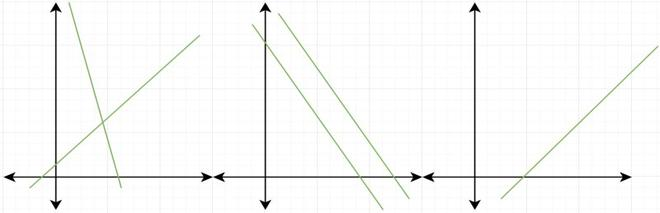(From left to right) Unique Solution, No Solution, and Infinitely many Solutions.

Question 1: Find the intersection between two lines given below:

3x + 4y = 12

x + y = 3

Such a system is solved using the substitution method.

x = 3 – y

Putting this value in the equation 1,

3(3 – y) + 4y = 12

9 – 3y + 4y = 12

y = 3

So, x = 0,

Thus, the solution is (0,3).

Question 2: Find the solution of the two lines.

x + y = 5

3x + 3y = 15